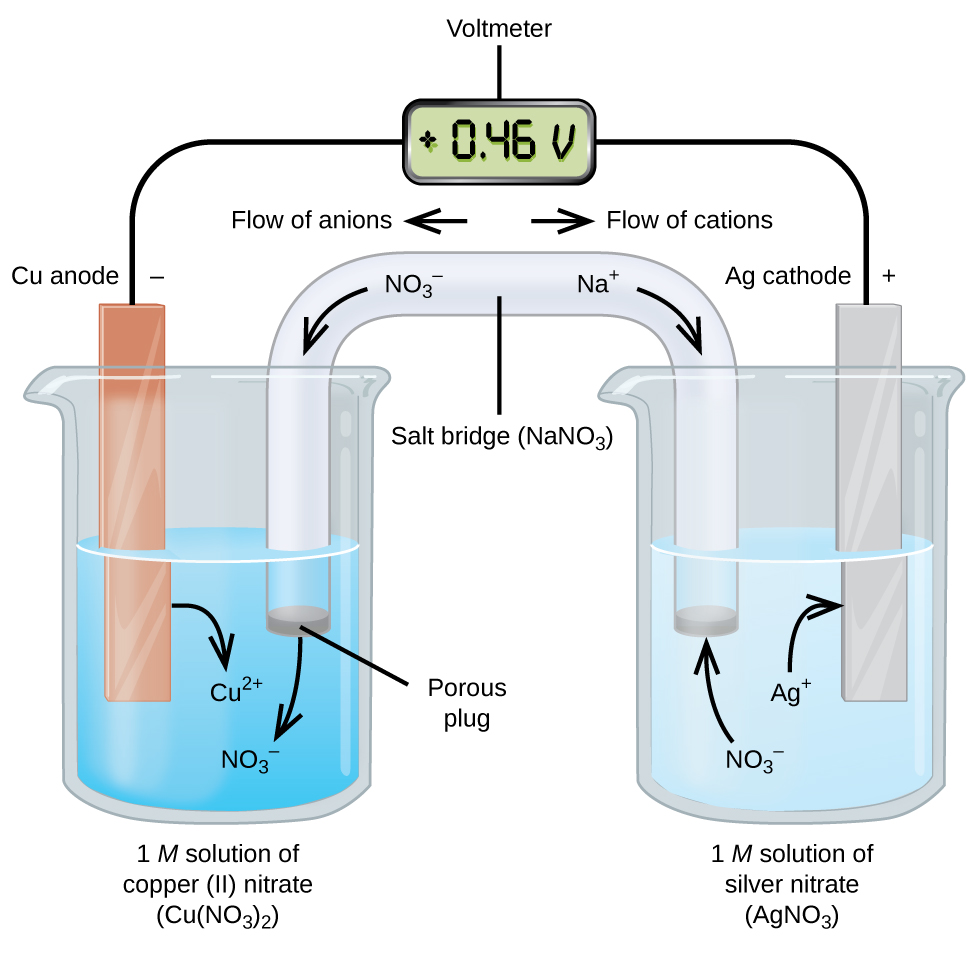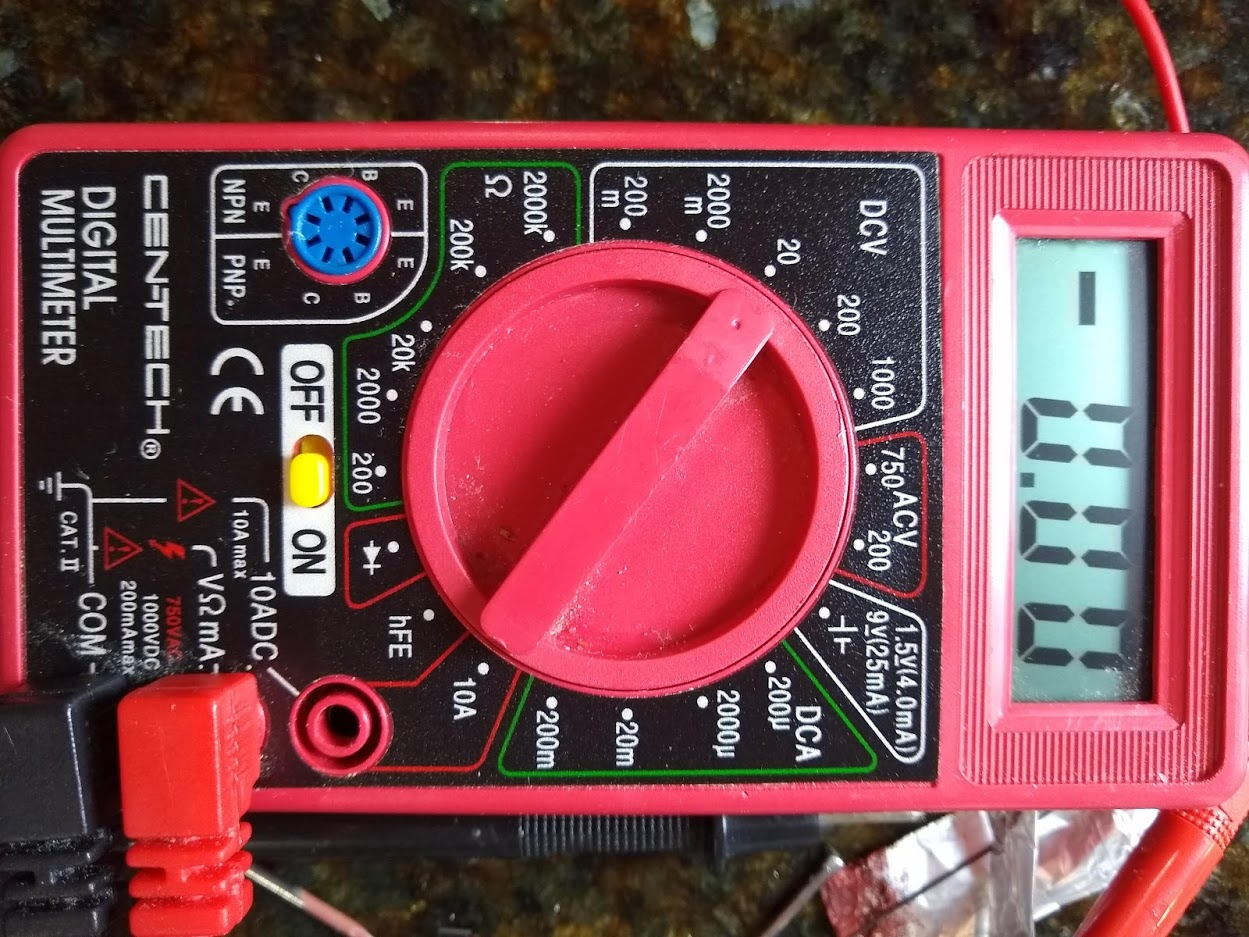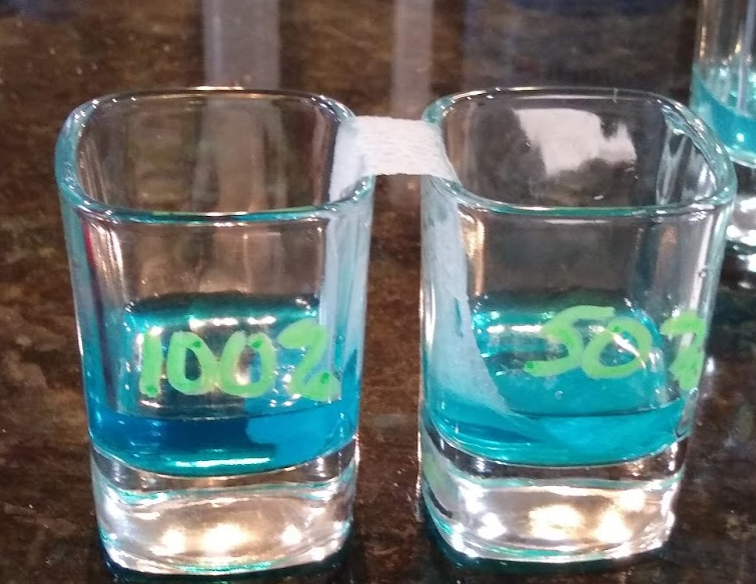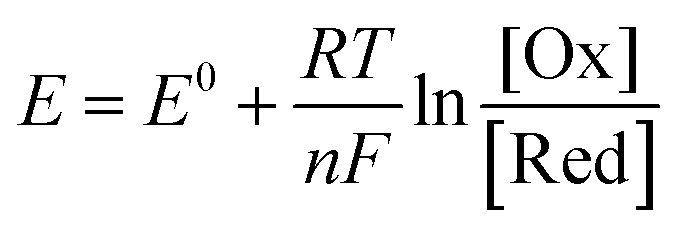Posted: May 17th, 2021

# Complete lab report | Chemistry homework help

## Introduction

This lab will require the student to create solutions of iron and aluminium by using electrolysis. Students will then observe the voltage created in a number of galvanic cells and create a reactivity order.

## Materials

• 9V battery
• Alligator clips * may have come with multimeter / voltmeter
• Aluminum foil
• Cups / Beakers – 7 – regardless of vessels used, they are called “beakers” in this lab
• CuSO4  – 30mL 1M CuSO4 solution or 7.5g solid CuSO4
• Finish nails – 5 *Some metal paper clips may work
• Graphite pieces – 8 – for mechanical pencils (something like this – the size isn’t relevant for this lab but you will need several sticks. If possible, choose a size for a pencil you already own)
• NaCl – 3-5g (table salt)
• Paper towel
• Pen for labeling cups / beakers
• Scissors
• Voltmeter / Multimeter (HarborFreight \$6.40, Amazon \$10.26, Walmart \$8.09, AliExpress (choose EMS ePacket it will take about 2 weeks) \$5.04
• Water – tap water

#### Galvanic Cells

A galvanic cell is a spontaneous production of a redox reaction. This is an electrochemical cell that will be demonstrated in the lab. A galvanic cell has an anode (oxidation reaction) and a cathode (reduction reaction).  The electrons flow through a salt bridge which does not react with other ions in solution.The salt bridge could be a paper towel or filter paper soaked in an ionic solution or it can be a porous disk between the anode and cathode chambers.

Each half reaction has a standard reduction potential that can be looked up on a table. The standard reduction potentials can be used to calculate the cell potential, in Volts, of a galvanic cell.

Eocell = Eocathode – Eoanode

For a cell that is made up of Zinc and Cobalt, the half reactions and their standard reduction potentials are:

Zn2+(aq) + 2e- →  Zn(s)  Eo = –  0.76V

Co2+(aq) + 2e- →  Co(s)  Eo = –  0.28V

Since cobalt has a more positive reduction potential, it will be the reduction reaction at the cathode. Zinc will be the oxidation reaction at the anode. The Eocell can then be calculated as:

Eocell = Eocathode – Eoanode

Eocell = EoCo – EoZn

Eocell = (-0.28) – (-0.76)

Eocell = +0.48V

### Nernst

Calculating redox potentials and determining spontaneity in non-standard conditions requires the use of the Nernst equation. (at 25 oC the following equation applies)

E = Eo – (0.0592V/n) logQ

Recall that Q is determined by the concentration of the products over the concentration of reactants. For this course, you should have only one species for each of the products and the reactants.

For example, What is the potential of a cell made up of Zn/Zn2+ and Cu/Cu2+ half-cells at 25oC if [Zn2+] = 2.5M and the [Cu2+]= 0.25M?

First step is to determine the reaction equation by looking at the standard reduction potentials to determine which species is reducing and which is oxidation.

Zn2+ + 2e- → Zn   Eo = -0.76

Cu2+ + 2e- → Cu   Eo = +0.34

Copper is the cathode because it is more positive. Now calculate the Eo.

Eocell = Eocathode – Eoanode

Eocell = EoCu – EoZn

Eocell = (0.34) – (-0.76)

Eocell = 1.10V

There are 2 electrons being transferred, n=2. Complete the Nernst Equation.

E = Eo – (0.0592V/n) log([Zn2+]/[ Cu2+])

E = 1.10 – (0.0592V/2) log(2.5M/0.25M)

E = 1.10 – 0.0296V

E = 1.07 V

### Concentration Cell

A concentration cell is a galvanic cell that is composed of the same species, but in different concentrations. For example, a cell composed of 2 zinc electrodes with one cell holding 0.10M zinc sulfate and the other holding 1.0M zinc sulfate can be calculated using a form of the Nernst equation as well.

E = Eo – (0.0592V/n) log([Zn2+]dilute/[ Zn2+]concentrated)

E = 0V – (0.0592V/2) log(0.1/1.0)

E = -0.030V

Electrolysis will be used to create solutions of iron and aluminum. Electrolysis is the use of electricity to force a reaction that otherwise is nonspontaneous.

Galvanic cells will then be set up and studied.

A Concentration cell of CuSO4 will also be studied.

### Familiarize yourself with voltmeter and set it up1. Prepare the Voltmeter or Multimeter (referred to as voltmeter).
2. Insert batteries, if needed.
3. Insert the leads appropriately and turn the dial to 20V. This is the setting that will display volts. Turn the voltmeter on.
4. Test the battery that will be used later in the lab by placing the red lead on the positive terminal of the 9V battery and the black lead on the negative side.
5. Verify that the display shows approximately 9V.
6. If the display shows less than 6V, get a new battery and verify the display again.

### Make solutions and anodes

When making these solutions, be sure to record your observations for the Discussion section.

1. NaCl solution
2. Tare a small beaker on the scale.
3. Mass approximately 4g of table salt into the beaker.
4. Add approximately 125mL of clean tap water to the beaker.
5. This is the NaCl solution
6. Graphite Electrodes
7. Gather mechanical pencil “lead” refills.
8. Snap approximately in half to create 12 pieces that are approximately 1 inch long.
9. These are the Graphite Electrodes.
10. CuSO4 Solution from solid CuSO4
11. Tare a small beaker on the scale.
12. Mass approximately 8g of CuSO4  into the beaker.
13. Add approximately 40mL of NaCl solution to the beaker.
14. This is the CuSO4 solution
15. CuSO4 solution – purchased as 1M CuSO4
16. Measure 40mL of premade 1M CuSO4 in a clean beaker.
17. Add a heavy pinch of salt to the solution.
18. This is the CuSO4 solution
19. Cu Electrodes
20. Measure approximately 10mL of CuSO4 solution into a clean beaker or into the 10mL graduated flask.
21. Place 1 Graphite electrode in the red alligator clip.
22. Place 1 Graphite electrode in the black alligator clip.
23. Attach the red alligator clip to the positive side of a 9V battery.
24. Attach the black alligator clip to the negative side of a 9V battery.
25. Insert the electrodes into measured CuSO4 solution prepared and measured in (a) of the Cu electrode section.
26. Allow this to react for approximately 1 minute.
27. Make sure to submerge the electrode on the black alligator clip as much as possible without getting the alligator clip wet.
28. Place the electrode that is now coated in red copper aside.
29. This is the Cu electrode.
30. Repeat b-i until there are 3 Cu electrodes prepared.
31. Al Electrodes
32. Cut Aluminum foil into 5 pieces approximately 1cm x 6cm.
33. These are the Al Electrodes.
34. Al3+ Solution
35. Measure 30mL of NaCl solution into a clean beaker.
36. Place 1 Aluminum electrode in the red alligator clip.
37. Place 1 Graphite electrode in the black alligator clip.
38. Attach the red alligator clip to the positive side of a 9V battery.
39. Attach the black alligator clip to the negative side of a 9V battery.
40. Insert the electrodes into the NaCl solution prepared and measured in (a) of the Al3+ section.
41. Allow this to react for approximately 3 minutes.
42. Dispose of both electrodes used.
43. This is the Al3+ solution.
44. Fe Electrodes
45. Gather 5 finish nails.
46. These are the Fe Electrodes.
47. Fe2+ Solution
48. Measure 30mL of NaCl solution into a clean beaker.
49. Place 1 Fe electrode in the red alligator clip.
50. Place 1 Graphite electrode in the black alligator clip.
51. Attach the red alligator clip to the positive side of a 9V battery.
52. Attach the black alligator clip to the negative side of a 9V battery.
53. Insert the electrodes into the NaCl solution prepared and measured in (a) of the Fe2+ section.
54. Allow this to react for approximately 2 minutes.
55. Dispose of both electrodes used.
56. This is the Fe2+ solution.
57. Take a picture of all of your prepared solutions and electrodes.

### Testing Reactivity

#### Solids in Liquids

1. Attach one side of the red alligator clip lead to the red voltmeter lead.
2. Attach one side of the black alligator clip lead to the black voltmeter lead.
3. Create a data table in the Results section of your lab report document to record all of the observed voltages.
4. Set up and record the voltage readings (highest viewed voltage during about 1 minute) of the following combinations. The solid metal electrode should be in the black alligator clip. There should be a fresh graphite electrode in the red alligator clip for each combination. Use a new metal electrode for each test. For the Fe electrodes, it is possible that you will need to briskly rub the surface of the electrode with a rough paper towel to remove the oxide layer. If you do not get expected readings, try to clean the electrode and retry the voltage readings.
5. Record any physical observations as well as the voltage.

Fe2+ solution

Al3+ solution

CuSO4 solution

Fe electrode

Al electrode

Cu electrode

#### Liquids and Liquids

1. Create a data table in the Results section of your lab report document to record all of the observed voltages.
2. Measure 10mL of CuSO4 solution into a clean beaker. This is the 100% solution.
3. Measure 5mL of NaCl solution into the 10mL graduated cylinder. Add 5mL of CuSO4 solution to the graduated cylinder. Pour the solution into a clean beaker. This is the 50% solution.
4. Measure 9mL of NaCl solution into the 10mL graduated cylinder. Add 1mL of CuSO4 solution to the graduated cylinder. Pour the solution into a clean beaker. This is the 10% solution.
5. Make 3 salt bridges
6. Cut strips of paper towel that will be long enough to touch the liquid level of two solutions when the beakers are next to one another.
7. Dip these paper towel pieces into the NaCl solution and gently squeeze the excess solution out.
8. Set these ion soaked paper towels aside.
9. These are the salt bridges.
10. Place two beakers next to one another and drape 1 salt bridge over the edge of the beakers so that one side is in the 100% solution and the other side of the salt bridge is in the 50% solution.1. Insert clean graphite electrodes in each alligator clip.
2. Place the graphite electrode attached to the black lead in the more concentrated solution. The graphite electrode with the red lead should be inserted into the less concentrated solution.
3. Without touching the salt bridge to the electrode, record the voltage reading (instantaneous high read allowing for about 1 minute of stabilization time) of the concentration cell.
4. Dispose of the salt bridge. Set the graphite electrodes near each solution used.
5. Repeat the above steps 6-9 for the other combinations; 100% | 10% & 50% | 10%

#### Concentration Cell Data TreatmentThe Nernst equation will be applied.

1. Calculate the Eo of the cell. (hint: the oxidation and reduction equations are likely to be equal and opposite one another! This is not a trick question.)
2. Determine n for this cell. (n = moles of electrons being transferred)
3. Calculate RT/F, the constant part. T should be standard room temperature. Either insert an actual reading or 298K.
4. The concentrations are relative. Use 100, 50, 10.  Which is the one in the numerator? The one that produces the sign you saw on your voltmeter.

### Expert paper writers are just a few clicks away

Place an order in 3 easy steps. Takes less than 5 mins.

## Calculate the price of your order

You will get a personal manager and a discount.
We'll send you the first draft for approval by at
Total price:
\$0.00